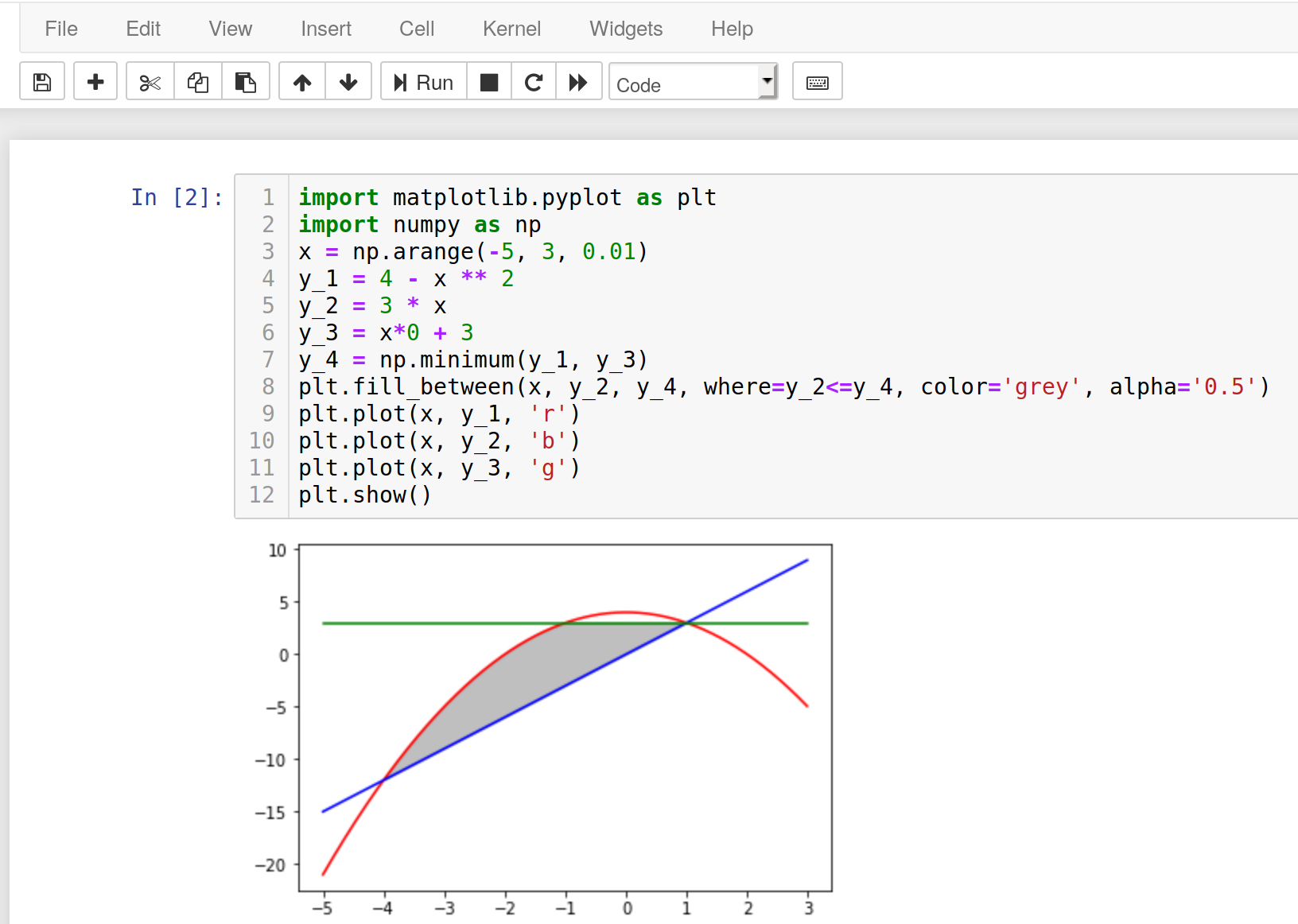# Field between 3 functions

What need to change in that code because it doesn't work? In python it works good but in Sage show error.

import matplotlib.pyplot as plt
import numpy as np
x = np.arange(-5, 3, 0.01)
y_1 = 4 - x ** 2
y_2 = 3 * x
y_3 = x*0 + 3
y_4 = np.minimum(y_1, y_3)
plt.fill_between(x, y_2, y_4, where=y_2<=y_4, color='grey', alpha='0.5')
plt.plot(x, y_1, 'r')
plt.plot(x, y_2, 'b')
plt.plot(x, y_3, 'g')
plt.show()


In Notebook, Sage 8.9, Ubuntuedit retag close merge delete